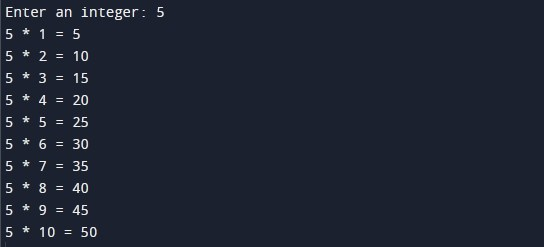C Program To Print Multiplication Table Of Given Number

In this tutorial you will learn about the C Program To Print Multiplication Table Of Given Number and its application with practical example.

In this tutorial, we will learn to create a program to generate and print multiplication table of given number using for loop In C programming language.

Prerequisites

Before starting with this tutorial we assume that you are best aware of the following C programming topics:

• C Operators
• C Variables
• C Input Output
• C for loop

Program to Generate Multiplication Table Of a Number

In this program we will generate multiplication table of a number entered by the user. We would first declared and initialized the required variables. Next, we would prompt user to input an integer number to print multiplication table. Later in the program we will generate and print multiplication table of that number.

Output:-In the above program, we have first declared and initialized a set variables required in the program.

• num = it holds the an integer number value
• i = iterator used in for loop

In the next statement user will be prompted to enter an integer number value. This will be assigned to variable ‘num’. Next, we will generate the multiplication table of the number using c for loop. Now, we will print the multiplication table of the integer number entered by user.

In this tutorial we have learn about the C Program To Print Multiplication Table Of Given Number and its application with practical example. I hope you will like this tutorial.